Most Affordable JEE | NEET | 8,9,10 Preparation by Kota's Top IITian Doctor Faculties

# NCERT Solutions for Class 11 Chemistry chapter 10 The s-block Elements PDF - eSaral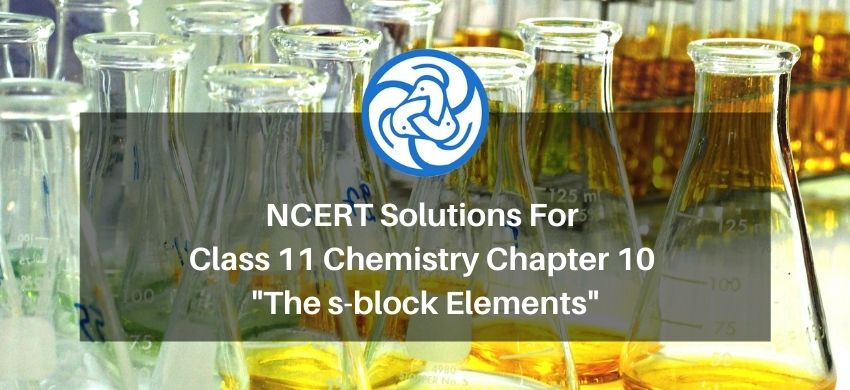`

# NCERT Solutions for Class 11 Chemistry chapter 10 The s-block Elements PDF

Hey, are you a class 11 student and looking for ways to download NCERT Solutions for Class 11 Chemistry chapter 10 The s-block Elements PDF? If yes. Then read this post till the end.

In this article, we have listed NCERT Solutions for Class 11 Chemistry chapter 10 The s-block Elements in PDF that are prepared by Kota’s top IITian’s Faculties by keeping Simplicity in mind.

If you want to learn and understand class 11 Chemistry chapter 10 "The s-block Elements" in an easy way then you can use these solutions PDF.

NCERT Solutions helps students to Practice important concepts of subjects easily. Class 11 Chemistry solutions provide detailed explanations of all the NCERT questions that students can use to clear their doubts instantly.

If you want to score high in your class 11 Chemistry Exam then it is very important for you to have a good knowledge of all the important topics, so to learn and practice those topics you can use eSaral NCERT Solutions.

So, without wasting more time Let’s start.

### Download NCERT Solutions for Class 11 Chemistry chapter 10 The s-block Elements PDF

Question 1: What are the common physical and chemical features of alkali metals?

Solution Physical properties of alkali metals:

(1) They are quite soft and can be cut easily. Sodium metal can be easily cut using a knife.

(2) They are light coloured and are mostly silvery white in appearance.

(3) They have low density because of the large atomic sizes. The density increases down the group from $\mathrm{Li}$ to $\mathrm{Cs}$. The only exception to this is $\mathrm{K}$, which has lower density than Na.

(4) The metallic bonding present in alkali metals is quite weak. Therefore, they have low melting and boiling points.

(5) Alkali metals and their salts impart a characteristic colour to flames. This is because the heat from the flame excites the electron present in the outermost orbital to a high energy level. When this excited electron reverts back to the ground state, it emits excess energy as radiation that falls in the visible region.

(6) They also display photoelectric effect. When metals such as $\mathrm{Cs}$ and $\mathrm{K}$ are irradiated with light, they lose electrons.

Chemical properties of alkali metals:

Alkali metals are highly reactive due to their low ionization enthalpy. As we move down the group, the reactivity increases.

(1) They react with water to form respective oxides or hydroxides. As we move down the group, the reaction becomes more and more spontaneous.

(2) They react with water to form their respective hydroxides and dihydrogens. The general reaction for the same is given as

$2 \mathrm{M}+2 \mathrm{H}_{2} \mathrm{O} \longrightarrow 2 \mathrm{M}^{+}+2 \mathrm{OH}^{\ominus}+\mathrm{H}_{2}$

(3) They react with dihydrogen to form metal hydrides. These hydrides are ionic solids and have high melting points.

$2 \mathrm{M}+\mathrm{H}_{2} \longrightarrow 2 \mathrm{M}^{+} \mathrm{H}^{-}$

(4) Almost all alkali metals, except Li, react directly with halogens to form ionic halides.

$2 \mathrm{M}+\mathrm{Cl}_{2} \longrightarrow 2 \mathrm{MCl}$

$(\mathrm{M}=\mathrm{Li}, \mathrm{K}, \mathrm{Rb}, \mathrm{Cs})$

Since $\mathrm{Li}^{+}$ ion is very small in size, it can easily distort the electron cloud around the negative halide ion. Therefore, lithium halides are covalent in nature.

(5) They are strong reducing agents. The reducing power of alkali metals increases on moving down the group. However, lithium is an exception. It is the strongest reducing agent among the alkali metals. It is because of its high hydration energy.

(6) They dissolve in liquid ammonia to form deep blue coloured solutions. These solutions are conducting in nature.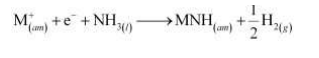In a highly concentrated solution, the blue colour changes to bronze and the solution becomes diamagnetic.

Question 2: Discuss the general characteristics and gradation in properties of alkaline earth metals.

Solution. General characteristics of alkaline earth metals are as follows:

(i) The general electronic configuration of alkaline earth metals is [noble gas] $n s^{2}$.

(ii) These metals lose two electrons to acquire the nearest noble gas configuration. Therefore, their oxidation state is $+2$.

(iii) These metals have atomic and ionic radii smaller than that of alkali metals. Also, whenmoved down the group, the effective nuclear charge decreases and this causes an increase in their atomic radii and ionic radii.

(iv) Since the alkaline earth metals have large size, their ionization enthalpies are found to be fairly low. However, their first ionization enthalpies are higher than the corresponding group 1 metals.

(v) These metals are lustrous and silvery white in appearance. They are relatively less soft as compared to alkali metals.

(vi)Atoms of alkaline earth metals are smaller than that of alkali metals. Also, they have two valence electrons forming stronger metallic bonds. These two factors cause alkaline earth metals to have high melting and boiling points as compared to alkali metals.

(vii) They are highly electropositive in nature. This is due to their low ionization enthalpies. Also, the electropositive character increases on moving down the group from Be to Ba.

(viii) Ca, Sr, and Ba impart characteristic colours to flames.

Ca - Brick red

Sr - Crimson red

Ba - Apple green

In Be and $\mathrm{Mg}$, the electrons are too strongly bound to be excited.

Hence, these do not impart any colour to the flame.

The alkaline earth metals are less reactive than alkali metals and their reactivity increases

on moving down the group.

Chemical properties of alkaline earth metals are as follows:

(i) Reaction with air and water: Be and $\mathrm{Mg}$ are almost inert to air and water because of the formation of oxide layer on their surface.

(a) Powdered Be burns in air to form $\mathrm{BeO}$ and $\mathrm{Be}_{3} \mathrm{~N}_{2}$.

(b) Mg, being more electropositive, burns in air with a dazzling sparkle to form $\mathrm{MgO}$ and $\mathrm{Mg}_{3} \mathrm{~N}_{2}$

(c) $\mathrm{Ca}, \mathrm{Sr}$, and Ba react readily with air to form respective oxides and nitrides.

(d) Ca, Ba, and Sr react vigorously even with cold water.

(ii) Alkaline earth metals react with halogens at high temperatures to form halides.

$\mathrm{M}+\mathrm{X}_{2} \longrightarrow \mathrm{MX}_{2}(\mathrm{X}=\mathrm{F}, \mathrm{Cl}, \mathrm{Br}, \mathrm{I})$

(iii) All the alkaline earth metals, except Be, react with hydrogen to form hydrides.

(iv) They react readily with acids to form salts and liberate hydrogen gas.

$\mathrm{M}+2 \mathrm{HCl} \longrightarrow \mathrm{MCl}_{2}+\mathrm{H}_{2(g)} \uparrow$

(v) They are strong reducing agents. However, their reducing power is less than that of alkali metals. As we move down the group, the reducing power increases.

(vi) Similar to alkali metals, the alkaline earth metals also dissolve in liquid ammonia to give deep blue coloured solutions.

$\mathrm{M}+(x-y) \mathrm{NH}_{3} \longrightarrow\left[\mathrm{M}\left(\mathrm{NH}_{3}\right)_{s}\right]^{+2}+2\left[\mathrm{e}\left(\mathrm{NH}_{3}\right)_{y}\right]^{-}$

Solution. Alkali metals include lithium, sodium, potassium, rubidium, cesium, and francium. These metals have only one electron in their valence shell, which they lose easily, owing to their mlow ionization energies. Therefore, alkali metals are highly reactive and are not found in nature in their elemental state.

Question 4: Find the oxidation state of sodium in Na2O2.

Solution. Let the oxidation state of Na be $x$.

The oxidation state of oxygen, in case of peroxides, is –1.

Therefore,

$2(x)+2(-1)=0$

$2 x-2=0$

$2 x=2$

$x=+1$

Therefore, the oxidation sate of sodium is +1.

Question 5: Explain why is sodium less reactive than potassium?

Solution. In alkali metals, on moving down the group, the atomic size increases and the effective nuclear charge decreases. Because of these factors, the outermost electron in potassium can be lost easily as compared to sodium. Hence, potassium is more reactive than sodium.

Question 6: Compare the alkali metals and alkaline earth metals with respect to

(i) ionization enthalpy

(ii) basicity of oxides and

(iii) solubility of hydroxides.

Solution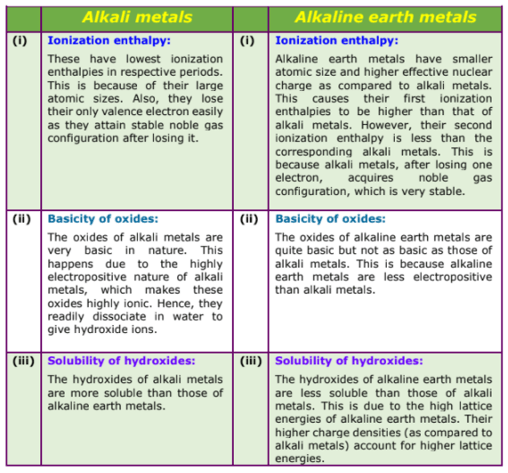Question 7: In what ways lithium shows similarities to magnesium in its chemical behavior?

Solution. Similarities between lithium and magnesium are as follows:

(i) Both Li and Mg react slowly with cold water.

(ii) The oxides of both Li and Mg are much less soluble in water and their hydroxides decompose at high temperature.

$2 \mathrm{LiOH}$ heat $\longrightarrow \mathrm{Li}_{2} \mathrm{O}+\mathrm{H}_{2} \mathrm{O}$

$\mathrm{Mg}(\mathrm{OH})_{2} \stackrel{\text { hest }}{\longrightarrow} \mathrm{MgO}+\mathrm{H}_{2} \mathrm{O}$

(iii) Both Li and Mg react with N2 to form nitrides.

$6 \mathrm{Li}+\mathrm{N}_{2} \stackrel{\text { hett }}{\longrightarrow} 2 \mathrm{Li}_{3} \mathrm{~N}$

$3 \mathrm{Mg}+\mathrm{N}_{2}$ \mathrm{ heit } ${\longrightarrow} \mathrm{Mg}_{3} \mathrm{~N}_{2}$

(iv) Neither Li nor Mg form peroxides or superoxides.

(v) The carbonates of both are covalent in nature. Also, these decompose on heating.

$\mathrm{Li}_{2} \mathrm{CO}_{3}$ heat $\mathrm{Li}_{2} \mathrm{O}+\mathrm{CO}_{2}$

$\mathrm{MgCO}_{3} \stackrel{\text { beut }}{\longrightarrow} \mathrm{MgO}+\mathrm{CO}_{2}$

(vi) Li and Mg do not form solid bicarbonates.

(vii) Both LiCl and MgCl2 are soluble in ethanol owing to their covalent nature.

(viii) Both LiCl and MgCl2 are deliquescent in nature. They crystallize from aqueous solutions as hydrates, for example,

$\mathrm{LiCl} \cdot 2 \mathrm{H}_{2} \mathrm{O}$ and $\mathrm{MgCl}_{2} \cdot 8 \mathrm{H}_{2} \mathrm{O}$

Question 8: Explain why alkali and alkaline earth metals cannot be obtained by chemical reduction methods?

Solution In the process of chemical reduction, oxides of metals are reduced using a stronger reducing agent. Alkali metals and alkaline earth metals are among the strongest reducing agents and the reducing agents that are stronger than them are not available.

Therefore, they cannot be obtained by chemical reduction of their oxides.

Question 9: Why are potassium and cesium, rather than lithium used in photoelectric cells?

Solution. All the three, lithium, potassium, and cesium, are alkali metals. Still, K and Cs are used in the photoelectric cell and not Li. This is because as compared to Cs and K, Li is smaller in size and therefore, requires high energy to lose an electron. While on the other hand, K and Cs have low ionization energy. Hence, they can easily lose electrons. This property of K and Cs is utilized in photoelectric cells.

Question 10: When an alkali metal dissolves in liquid ammonia the solution can acquire different colours. Explain the reasons for this type of colour change.

Solution. When an alkali metal is dissolved in liquid ammonia, it results in the formation of a deep blue coloured solution.

$\mathrm{M}+(x+y) \mathrm{NH}_{3} \longrightarrow \mathrm{M}^{+}\left(\mathrm{NH}_{3}\right)_{x}+\mathrm{e}^{-1}\left(\mathrm{NH}_{3}\right)_{y}$

The ammoniated electrons absorb energy corresponding to red region of visible light. Therefore, the transmitted light is blue in colour.

At a higher concentration (3 M), clusters of metal ions are formed. This causes the solution to attain a copper–bronze colour and a characteristic metallic lustre.

Question 11: Beryllium and magnesium do not give colour to flame whereas other alkaline earth metals do so. Why?

Solution. When an alkaline earth metal is heated, the valence electrons get excited to a higher energy level. When this excited electron comes back to its lower energy level, it radiates energy, which belongs to the visible region. Hence, the colour is observed. In Be and $\mathrm{Mg}$, the electrons are strongly bound. The energy required to excite these electrons is very high. Therefore, when the electron reverts back to its original position, the energy released does not fall in the visible region. Hence, no colour in the flame is seen.

Question 12: Discuss the various reactions that occur in the Solvay process.

Solution. Solvay process is used to prepare sodium carbonate.

When carbon dioxide gas is bubbled through a brine solution saturated with ammonia,

sodium hydrogen carbonate is formed. This sodium hydrogen carbonate is then converted to sodium carbonate.

Step 1: Brine solution is saturated with ammonia.

$2 \mathrm{NH}_{3}+\mathrm{H}_{2} \mathrm{O}+\mathrm{CO}_{2} \longrightarrow\left(\mathrm{NH}_{4}\right)_{2} \mathrm{CO}_{3}$

This ammoniated brine is filtered to remove any impurity.

Step 2: Carbon dioxide is reacted with this ammoniated brine to result in the formation of insoluble sodium hydrogen carbonate.

$\mathrm{NH}_{3}+\mathrm{H}_{2} \mathrm{O}+\mathrm{CO}_{2} \longrightarrow \mathrm{NH}_{4} \mathrm{HCO}_{3}$

$\mathrm{NaCl}+\mathrm{NH}_{4} \mathrm{HCO}_{3} \longrightarrow \mathrm{NH}_{4} \mathrm{HCO}_{3} \downarrow+\mathrm{NH}_{4} \mathrm{Cl}$

Step 3: The solution containing crystals of $\mathrm{NaHCO}_{3}$ is filtered to obtain $\mathrm{NaHCO}_{3}$.

Step 4: $\mathrm{NaHCO}_{3}$ is heated strongly to convert it into $\mathrm{NaHCO}_{3}$.

$2 \mathrm{NaHCO}_{3} \longrightarrow \mathrm{Na}_{2} \mathrm{CO}_{3}+\mathrm{CO}_{2}+\mathrm{H}_{2} \mathrm{O}$

Step 5: To recover ammonia, the filtrate (after removing $\mathrm{NaHCO}_{3}$ ) is mixed with $\mathrm{Ca}(\mathrm{OH})_{2}$ and heated.

$\mathrm{Ca}(\mathrm{OH})_{2}+2 \mathrm{NH}_{4} \mathrm{Cl} \longrightarrow \mathrm{LNH}_{3}+2 \mathrm{H}_{2} \mathrm{O}+\mathrm{CaCl}_{2}$

The overall reaction taking place in Solvay process is

$2 \mathrm{NaCl}+\mathrm{CaCO}_{3} \longrightarrow \mathrm{Na}_{2} \mathrm{CO}_{3}+\mathrm{CaCl}_{2}$

Question 13: Potassium carbonate cannot be prepared by Solvay process. Why?

Solution. Solvay process cannot be used to prepare potassium carbonate. This is because unlike sodium bicarbonate, potassium bicarbonate is fairly soluble in water and does not precipitate out.

Question 14: Why is Li2CO3 decomposed at a lower temperature whereas Na2CO3 at higher temperature?

Solution As we move down the alkali metal group, the electropositive character increases. This causes an increase in the stability of alkali carbonates. However, lithium carbonate is not so stable to heat. This is because lithium carbonate is covalent. Lithium ion, being very small in size, polarizes a large carbonate ion, leading to the formation of more stable lithium oxide.

$\mathrm{Li}_{2} \mathrm{CO}_{3} \stackrel{\triangle}{\longrightarrow} \mathrm{Li}_{2} \mathrm{O}+\mathrm{CO}_{2}$

Therefore, lithium carbonate decomposes at a low temperature while a stable sodium carbonate decomposes at a high temperature.

Question 15: Compare the solubility and thermal stability of the following compounds of the alkali metals with those of the alkaline earth metals. (i) Nitrates (ii) Carbonates (iii) Sulphates.

Solution (i) Nitrates

Thermal stability

Nitrates of alkali metals, except LiNO $_{3}$, decompose on strong heating to form nitrites.

$2 \mathrm{KNO}_{3(s)} \longrightarrow 2 \mathrm{KNO}_{2(s)}+\mathrm{O}_{2(g)}$

$\mathrm{LiNO}_{3}$, on decomposition, gives oxide.

$2 \mathrm{LiNO}_{3(-)} \stackrel{\Delta}{\longrightarrow} \mathrm{Li}_{2} \mathrm{O}_{(s)}+2 \mathrm{NO}_{2(g)}+\mathrm{O}_{2(g)}$

Similar to lithium nitrate, alkaline earth metal nitrates also decompose to give oxides.

$2 \mathrm{Ca}\left(\mathrm{NO}_{3}\right)_{(x)} \stackrel{\Delta}{\longrightarrow} 2 \mathrm{CaO}_{(t)}+4 \mathrm{NO}_{2(g)}+\mathrm{O}_{2(g)}$

As we move down group 1 and group 2, the thermal stability of nitrate increases.

Solubility

Nitrates of both group 1 and group 2 metals are soluble in water.

(ii) Carbonates

Thermal stability

The carbonates of alkali metals are stable towards heat. However, carbonate of lithium, when heated, decomposes to form lithium oxide. The carbonates of alkaline earth metals also decompose on heating to form oxide and carbon dioxide.

$\mathrm{Na}_{2} \mathrm{CO}_{3} \stackrel{\Delta}{\longrightarrow}$ No effect

$\mathrm{Li}_{2} \mathrm{CO}_{3} \stackrel{\Delta}{\longrightarrow} \mathrm{Li}_{2} \mathrm{O}+\mathrm{CO}_{2}$

$\mathrm{MgCO}_{3} \stackrel{\Delta}{\longrightarrow} \mathrm{MgO}+\mathrm{CO}_{2}$

Solubility

Carbonates of alkali metals are soluble in water with the exception of $\mathrm{Li}_{2} \mathrm{CO}_{3}$. Also, the

solubility increases as we move down the group.

Carbonates of alkaline earth metals are insoluble in water.

(iii) Sulphates

Thermal stability

Sulphates of both group 1 and group 2 metals are stable towards heat.

Solubility

Sulphates of alkali metals are soluble in water. However, sulphates of alkaline earth metals

show varied trends.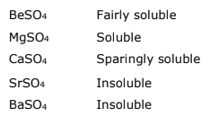In other words, while moving down the alkaline earth metals, the solubility of their sulphates decreases.

Question 16: Starting with sodium chloride how would you proceed to prepare

(i) sodium metal

(ii) sodium hydroxide

(iii) sodium peroxide

(iv) sodium carbonate?

Solution (i) Sodium can be extracted from sodium chloride by Downs process.

This process involves the electrolysis of fused $\mathrm{NaCl}(40 \%)$ and $\mathrm{CaCl}_{2}(60 \%)$ at a

temperature of $1123 \mathrm{~K}$ in Downs cell.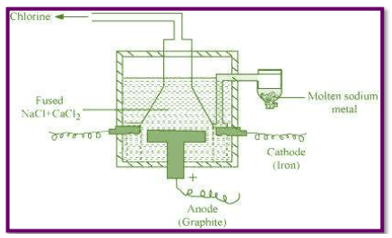Steel is the cathode and a block of graphite acts as the anode. Metallic Na and $\mathrm{Ca}$ are formed at cathode. Molten sodium is taken out of the cell and collected over kerosene.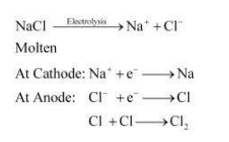(ii) Sodium hydroxide can be prepared by the electrolysis of sodium chloride. This is called Castner-Kellner process. In this process, the brine solution is electrolysed using a carbon anode and a mercury cathode.

The sodium metal, which is discharged at cathode, combines with mercury to form an amalgam.

Cathode: $\mathrm{Na}^{+}+\mathrm{e}^{-} \stackrel{\mathrm{Hg}_{\mathrm{g}}}{\longrightarrow} \mathrm{Na}-$ amalgam

Anode: $\mathrm{Cl}^{-} \longrightarrow \frac{1}{2} \mathrm{Cl}_{2}+\mathrm{e}^{-}$

(iii) Sodium peroxide

First, $\mathrm{NaCl}$ is electrolysed to result in the formation of Na metal (Downs process). This sodium metal is then heated on aluminium trays in air (free of $\mathrm{CO}_{2}$ ) to form its peroxide.

(iv) Sodium carbonate is prepared by Solvay process. Sodium hydrogen carbonate is precipitated in a reaction of sodium chloride and ammonium hydrogen carbonate.

$2 \mathrm{NH}_{3}+\mathrm{H}_{2} \mathrm{O}+\mathrm{CO}_{2} \longrightarrow\left(\mathrm{NH}_{4}\right)_{2} \mathrm{CO}_{3}$

$\left(\mathrm{NH}_{4}\right)_{2} \mathrm{CO}_{3}+\mathrm{H}_{2} \mathrm{O}+\mathrm{CO}_{2} \longrightarrow 2 \mathrm{NH}_{4} \mathrm{HCO}_{3}$

$\mathrm{NH}_{4} \mathrm{HCO}_{3}+\mathrm{NaCl} \longrightarrow \mathrm{NH}_{4} \mathrm{Cl}+\mathrm{NaHCO}_{3}$

These sodium hydrogen carbonate crystals are heated to give sodium carbonate.

$2 \mathrm{NaHCO}_{3} \longrightarrow \mathrm{Na}_{2} \mathrm{CO}_{3}+\mathrm{CO}_{2}+\mathrm{H}_{2} \mathrm{O}$

Question 17: What happens when (i) magnesium is burnt in air (ii) quick lime is heated with silica

(iii) chlorine reacts with slaked lime (iv) calcium nitrate is heated?

Solution. (i) Magnesium burns in air with a dazzling light to form $\mathrm{MgO}$ and $\mathrm{Mg}_{3} \mathrm{~N}_{2}$.

$2 \mathrm{Mg}+\mathrm{O}_{2} \stackrel{\text { Bumine }}{\longrightarrow} 2 \mathrm{MgO}$

$3 \mathrm{Mg}+\mathrm{N}_{2} \stackrel{\text { Buming }}{\longrightarrow} \mathrm{Mg}_{3} \mathrm{~N}_{2}$

(ii) Quick lime (CaO) combines with silica ( $\mathrm{SiO}_{2}$ ) to form slag.

$\mathrm{CaO}+\mathrm{SiO}_{2} \stackrel{\text { hatat }}{\longrightarrow} \mathrm{CaSiO}_{3}$

(iii) When chloride is added to slaked lime, it gives bleaching powder.

$\mathrm{Ca}(\mathrm{OH})_{2}+\mathrm{Cl}_{2} \stackrel{\triangle}{\longrightarrow} \mathrm{CaOCl}_{2}+\mathrm{H}_{2} \mathrm{O}$

Bleaching

powder

(iv) Calcium nitrate, on heating, decomposes to give calcium oxide.

$2 \mathrm{Ca}\left(\mathrm{NO}_{3}\right)_{2(s)} \stackrel{\triangle}{\longrightarrow} 2 \mathrm{CaO}_{(s)}+4 \mathrm{NO}_{2(g)}+\mathrm{O}_{2(g)}$

Question 18: Describe two important uses of each of the following:

(i) caustic soda

(ii) sodium carbonate

(iii) quicklime.

Solution. (i) Uses of caustic soda

(a) It is used in soap industry.

(b) It is used as a reagent in laboratory.

(ii) Uses of sodium carbonate

(a) It is generally used in glass and soap industry.

(b) It is used as a water softener.

(iii) Uses of quick lime

(a) It is used as a starting material for obtaining slaked lime.

(b) It is used in the manufacture of glass and cement.

Question 19: Draw the structure of

(i) $\mathrm{BeCl}_{2}$ (Solid) $\quad$

(ii) $\mathrm{BeCl}_{2}$ (Vapour).

Solution. (i) Structure of $\mathrm{BeCl}_{2}$ (solid)

$\mathrm{BeCl}_{2}$ exists as a polymer in condensed (solid) phase.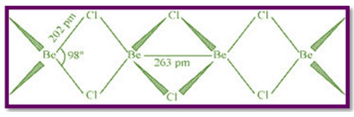(ii) In the vapour state, $\mathrm{BeCl}_{2}$ exists as a monomer with a linear structure.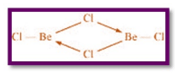Question 20: The hydroxides and carbonates of sodium and potassium are easily soluble in water while the corresponding salts of magnesium and calcium are sparingly soluble in water. Explain.

Solution. The atomic size of sodium and potassium is larger than that of magnesium and calcium. Thus, the lattice energies of carbonates and hydroxides formed by calcium and magnesium are much more than those of sodium and potassium. Hence, carbonates and hydroxides of sodium and potassium dissolve readily in water whereas those of calcium and magnesium are only sparingly soluble.

Question 21: Describe the importance of the following:

(i) limestone

(ii) cement

(iii) plaster of paris.

Solution. (i) Chemically, limestone is $\mathrm{CaCO}_{3}$.

Importance of limestone

(a) It is used in the preparation of lime and cement.

(b) It is used as a flux during the smelting of iron ores.

(ii) Chemically, cement is a mixture of calcium silicate and calcium aluminate.

Importance of cement

(a) It is used in plastering and in construction of bridges.

(b) It is used in concrete.

(iii) Chemically, plaster of Paris is $2 \mathrm{CaSO}_{4} \cdot \mathrm{H}_{2} \mathrm{O}$.

Importance of plaster of Paris

(a) It is used in surgical bandages.

(b) It is also used for making casts and moulds.

Question 22: Why are lithium salts commonly hydrated and those of the other alkali metal ions usually anhydrous?

Solution. Lithium is the smallest in size among the alkali metals. Hence, $\mathrm{Li}^{+}$ ion can polarize water molecules more easily than other alkali metals. As a result, water molecules get attached to lithium salts as water of crystallization. Hence, lithium salts such as trihydrated lithium chloride $\left(\mathrm{LiCl} \cdot 3 \mathrm{H}_{2} \mathrm{O}\right)$ are commonly hydrated. As the size of the ions increases, their polarizing power decreases. Hence, other alkali metal ions usually form anhydrous salts.

Question 23: Why is Lif almost insoluble in water whereas LiCl soluble not only in water but also in acetone?

Solution. LiF is insoluble in water. On the contrary, $\mathrm{LICl}$ is soluble not only in water, but also in acetone. This is mainly because of the greater ionic character of Lif as compared to LiCl. The solubility of a compound in water depends on the balance between lattice energy and hydration energy. Since fluoride ion is much smaller in size than chloride ion, the lattice energy of Lif is greater than that of LiCl. Also, there is not much difference between the hydration energies of fluoride ion and chloride ion. Thus, the net energy change during the dissolution of LiCl in water is more exothermic than that during the dissolution of LiF in water. Hence, low lattice energy and greater covalent character are the factors making LiCl soluble not only in water, but also in acetone.

Question 24: Explain the significance of sodium, potassium, magnesium and calcium in biological fluids.

Solution. Importance of sodium, potassium, magnesium, and calcium in biological fluids:

(i) Sodium (Na):

Sodium ions are found primarily in the blood plasma. They are also found in the interstitial fluids surrounding the cells.

(a) Sodium ions help in the transmission of nerve signals.

(b) They help in regulating the flow of water across the cell membranes.

(c) They also help in transporting sugars and amino acids into the cells.

(ii) Potassium (K):

Potassium ions are found in the highest quantity within the cell fluids.

(a) $\mathrm{K}$ ions help in activating many enzymes.

(b) They also participate in oxidising glucose to produce ATP.

(c) They also help in transmitting nerve signals.

(iii) Magnesium (Mg) and calcium (Ca):

Magnesium and calcium are referred to as macro-minerals. This term indicates their higher abundance in the human body system.

(a) Mg helps in relaxing nerves and muscles.

(b) $\mathrm{Mg}$ helps in building and strengthening bones.

(c) Mg maintains normal blood circulation in the human body system.

(d) Ca helps in the coagulation of blood

(e) Ca also helps in maintaining homeostasis.

Question 25: What happens when

(i) sodium metal is dropped in water?

(ii) sodium metal is heated in free supply of air ?

(iii) sodium peroxide dissolves in water ?

Solution (i) When Na metal is dropped in water, it reacts violently to form sodium hydroxide and hydrogen gas. The chemical equation involved in the reaction is:

$2 \mathrm{Na}_{(x)}+2 \mathrm{H}_{2} \mathrm{O}_{(n} \longrightarrow 2 \mathrm{NaOH}_{\text {(aq) }}+\mathrm{H}_{2(g)}$

(ii) On being heated in air, sodium reacts vigorously with oxygen to form sodium peroxide. The chemical equation involved in the reaction is:

$2 \mathrm{Na}_{(\omega)}+\mathrm{O}_{2(s)} \longrightarrow \mathrm{Na}_{2} \mathrm{O}_{2(n)}$

(iii) When sodium peroxide is dissolved in water, it is readily hydrolysed to form sodium hydroxide and water. The chemical equation involved in the reaction is:

$\mathrm{Na}_{2} \mathrm{O}_{2(\mathrm{x})}+2 \mathrm{H}_{2} \mathrm{O}_{(0} \longrightarrow 2 \mathrm{NaOH}_{(\omega)}+\mathrm{H}_{2} \mathrm{O}_{2(-(\alpha)}$

Question 26: Comment on each of the following observations:

(a) The mobilities of the alkali metal ions in aqueous solution are

$\mathrm{Li}^{+}<\mathrm{Na}^{+}<\mathrm{K}^{+}<\mathrm{Rb}^{+}<\mathrm{Cs}^{+}$

(b) Lithium is the only alkali metal to form a nitride directly.

(c) $\mathrm{E}^{\circ}$ for $\mathrm{M}^{2+}(\mathrm{aq})+2 \mathrm{e}^{-} \longrightarrow \mathrm{M}(\mathrm{s})$

(where $\mathrm{M}=\mathrm{Ca}, \mathrm{Sr}$ or $\mathrm{Ba}$ ) is nearly constant.

Solution. (a) On moving down the alkali group, the ionic and atomic sizes of the metals increase.

The given alkali metal ions can be arranged in the increasing order of their ionic sizes

as: $\quad \mathrm{Li}^{+}<\mathrm{Na}^{+}<\mathrm{K}^{+}<\mathrm{Rb}^{+}<\mathrm{Cs}^{+}$

Smaller the size of an ion, the more highly is it hydrated. Since $\mathrm{Li}^{+}$ is the smallest, it gets heavily hydrated in an aqueous solution. On the other hand, $\mathrm{Cs}^{+}$ is the largest and so it is the least hydrated. The given alkali metal ions can be arranged in the decreasing order of their hydrations as:

$\mathrm{Li}^{+}>\mathrm{Na}^{+}>\mathrm{K}^{+}>\mathrm{Rb}^{+}>\mathrm{Cs}^{+}$

Greater the mass of a hydrated ion, the lower is its ionic mobility. Therefore, hydrated $\mathrm{Li}^{+}$ is the least mobile and hydrated $\mathrm{Cs}^{+}$ is the most mobile. Thus, the given alkali metal ions can be arranged in the increasing order of their mobilities as:

$\mathrm{Li}^{+}<\mathrm{Na}^{+}<\mathrm{K}^{+}<\mathrm{Rb}^{+}<\mathrm{Cs}^{+}$

(b) Unlike the other elements of group 1, Li reacts directly with nitrogen to form lithium nitride. This is because $\mathrm{Li}^{+}$ is very small in size and so its size is the most compatible with the $\mathrm{N}^{3-}$ ion. Hence, the lattice energy released is very high. This energy also overcomes the high amount of energy required for the formation of the $\mathrm{N}^{3-}$ ion.

(c) Electrode potential $\left(E^{\circ}\right)$ of any $\mathrm{M}^{2+} / \mathrm{M}$ electrode depends upon three factors:

(i) Ionisation enthalpy

(ii) Enthalpy of hydration

(iii) Enthalpy of vaporization

The combined effect of these factors is approximately the same for $\mathrm{Ca}, \mathrm{Sr}$, and $\mathrm{Ba}$.

Hence, their electrode potentials are nearly constant.

Question 27: State as to why

(a) a solution of $\mathrm{Na}_{2} \mathrm{CO}_{3}$ is alkaline ?

(b) alkali metals are prepared by electrolysis of their fused chlorides?

(c) sodium is found to be more useful than potassium?

Solution. (a) When sodium carbonate is added to water, it hydrolyses to give sodium bicarbonate and sodium hydroxide (a strong base). As a result, the solution becomes alkaline.

$\mathrm{Na}_{2} \mathrm{CO}_{3}+\mathrm{H}_{2} \mathrm{O} \longrightarrow \mathrm{NaHCO}_{3}+\mathrm{NaOH}$

(b) It is not possible to prepare alkali metals by the chemical reduction of their oxides as they themselves are very strong reducing agents. They cannot be prepared by displacement reactions either (wherein one element is displaced by another). This is because these elements are highly electropositive. Neither can electrolysis of aqueous solutions be used to extract these elements. This is because the liberated metals react with water.

Hence, to overcome these difficulties, alkali metals are usually prepared by the electrolysis of their fused chlorides.

(c) Blood plasma and the interstitial fluids surrounding the cells are the regions where sodium ions are primarily found. Potassium ions are located within the cell fluids. Sodium ions are involved in the transmission of nerve signals, in regulating the flow of water across the cell membranes, and in transporting sugars and amino acids into the cells. Hence, sodium is found to be more useful than potassium.

Question 28: Write balanced equations for reactions between

(a) $\mathrm{Na}_{2} \mathrm{O}_{2}$ and water

(b) $\mathrm{KO}_{2}$ and water

(c) $\mathrm{Na}_{2} \mathrm{O}$ and $\mathrm{CO}_{2}$

Solution. (a) The balanced chemical equation for the reaction between $\mathrm{Na}_{2} \mathrm{O}_{2}$ and water is:

$2 \mathrm{Na}_{2} \mathrm{O}_{2(s)}+2 \mathrm{H}_{2} \mathrm{O}_{(i)} \longrightarrow 4 \mathrm{NaOH}_{(a q)}+\mathrm{O}_{2(a q)}$

(b) The balanced chemical equation for the reaction between $\mathrm{KO}_{2}$ and water is:

$2 \mathrm{KO}_{2(x)}+2 \mathrm{H}_{2} \mathrm{O}_{(\eta)} \longrightarrow 2 \mathrm{KOH}_{(\alpha a)}+\mathrm{H}_{2} \mathrm{O}_{2(\text { a })}+\mathrm{O}_{2(g)}$

(c) The balanced chemical equation for the reaction between $\mathrm{Na}_{2} \mathrm{O}$ and $\mathrm{CO}_{2}$ is:

$\mathrm{Na}_{2} \mathrm{O}_{(\mathrm{k})}+\mathrm{CO}_{2(g)} \longrightarrow \mathrm{Na}_{2} \mathrm{CO}_{3}$

Question 29: How would you explain the following observations?

(i) $\mathrm{BeO}$ is almost insoluble but $\mathrm{BeSO}_{4}$ in soluble in water,

(ii) $\mathrm{BaO}$ is soluble but $\mathrm{BaSO}_{4}$ is insoluble in water,

(iii) LiI is more soluble than KI in ethanol.

Solution. (i) $\mathrm{BeO}$ is almost insoluble in water and $\mathrm{BeSO}_{4}$ is soluble in water. $\mathrm{Be}^{2+}$ is a small cation with a high polarising power and $\mathrm{O}^{2-}$ is a small anion. The size compatibility of $\mathrm{Be}^{2+}$ and $\mathrm{O}^{2-}$ is high. Therefore, the lattice energy released during their formation is also very high. When BeO is dissolved in water, the hydration energy of its ions is not sufficient to overcome the high lattice energy. Therefore, BeO is insoluble in water. On the other hand, So $_{4}^{2-}$ ion is a large anion. Hence, $\mathrm{Be}^{2+}$ can easily polarize $\mathbf{S O}_{4}^{2-}$ ions, making $\mathrm{BeSO}_{4}$ unstable. Thus, the lattice energy of $\mathrm{BeSO}_{4}$ is not very high and so it is soluble in water.

(ii) $\mathrm{BaO}$ is soluble in water, but $\mathrm{BaSO}_{4}$ is not. $\mathrm{Ba}^{2+}$ is a large cation and $\mathrm{O}^{2-}$ is a small anion. The size compatibility of $\mathrm{Ba}^{2+}$ and $\mathrm{O}^{2-}$ is not high. As a result, BaO is unstable. The lattice energy released during its formation is also not very large. It can easily be overcome by the hydration energy of the ions. Therefore, $\mathrm{BaO}$ is soluble in water. In

$\mathrm{BaSO}_{4}, \mathrm{Ba}^{2+}$ and $\mathrm{SO}_{4}^{2-}$ are both large-sized. The lattice energy released is high. Hence, it is not soluble in water.

(iii) LiI is more soluble than $\mathrm{KI}$ in ethanol. As a result of its small size, the lithium ion has a higher polarising power than the potassium ion. It polarises the electron cloud of the iodide ion to a much greater extent than the potassium ion. This causes a greater covalent character in LiI than in KI. Hence, LiI is more soluble in ethanol.

Question 30: Which of the alkali metal is having least melting point?

(a) $\mathrm{Na}$

(b) $\mathrm{K}$

(c) $\mathrm{Rb}$

(d) $\mathrm{Cs}$

Solution. Atomic size increases as we move down the alkali group. As a result, the binding energies of their atoms in the crystal lattice decrease. Also, the strength of metallic bonds decreases on moving down a group in the periodic table. This causes a decrease in the melting point. Among the given metals, $\mathrm{Cs}$ is the largest and has the least melting point.

Question $31:$ Which one of the following alkali metals gives hydrated salts?

(a) Li

(b) $\mathrm{Na}$

(c) $\mathrm{K}$

(d) $\mathrm{Cs}$

Solution. Smaller the size of an ion, the more highly is it hydrated. Among the given alkali metals, $\mathrm{Li}$ is the smallest in size. Also, it has the highest charge density and highest polarizing power. Hence, it attracts water molecules more strongly than the other alkali metals. As a result, it forms hydrated salts such as $\mathrm{LiCl} .2 \mathrm{H}_{2} \mathrm{O}$. The other alkali metals are larger than Li and have weaker charge densities.

Hence, they usually do not form hydrated salts.

Question 32: Which one of the alkaline earth metal carbonates is thermally the most stable?

Solution. Thermal stability increases with the increase in the size of the cation present in the carbonate. The increasing order of the cationic size of the given alkaline earth metals is

$\mathrm{Mg}<\mathrm{Ca}<\mathrm{Sr}<\mathrm{Ba}$

Hence, the increasing order of the thermal stability of the given alkaline earth metal carbonates is

$\mathrm{MgCO}_{3}<\mathrm{CaCO}_{3}<\mathrm{SrCO}_{3}<\mathrm{BaCO}_{3}$